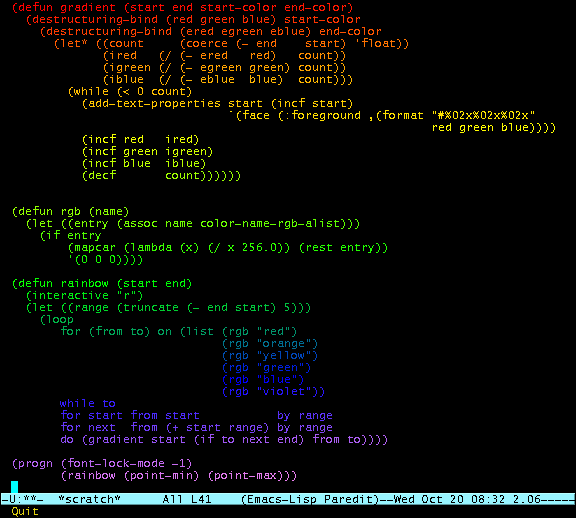# Playing with colors in emacs

The fundamental feature to set the color of characters in emacs are the text properties, namely, the face text property.

An important precondition is to disable font-locking, since this feature will override any colorizing you may be doing, usually soon enough that you won't even see the result of your settings.

```(add-text-properties start end
`(face (:foreground ,(format "#%02x%02x%02x" red green blue)))
``````
(require 'cl)

(defun gradient (start end start-color end-color)
(destructuring-bind (red green blue) start-color
(destructuring-bind (ered egreen eblue) end-color
(let* ((count     (coerce (- end    start) 'float))
(ired   (/ (- ered   red)   count))
(igreen (/ (- egreen green) count))
(iblue  (/ (- eblue  blue)  count)))
(while (< 0 count)
`(face (:foreground ,(format "#%02x%02x%02x"
red green blue))))
(incf red   ired)
(incf green igreen)
(incf blue  iblue)
(decf       count))))))

(defun rgb (name)
(let ((entry (assoc name color-name-rgb-alist)))
(if entry
(mapcar (lambda (x) (/ x 256.0)) (rest entry))
'(0 0 0))))

(defun rainbow (start end)
(interactive "r")
(let ((range (truncate (- end start) 5)))
(loop
for (from to) on (list (rgb "red")
(rgb "orange")
(rgb "yellow")
(rgb "green")
(rgb "blue")
(rgb "violet"))
while to
for start from start           by range
for next  from (+ start range) by range
do (gradient start (if to next end) from to))))

(progn (font-lock-mode -1)
(rainbow (point-min) (point-max)))

```

``` | Mirror on informatimago.com | Mirror on free.fr | ```